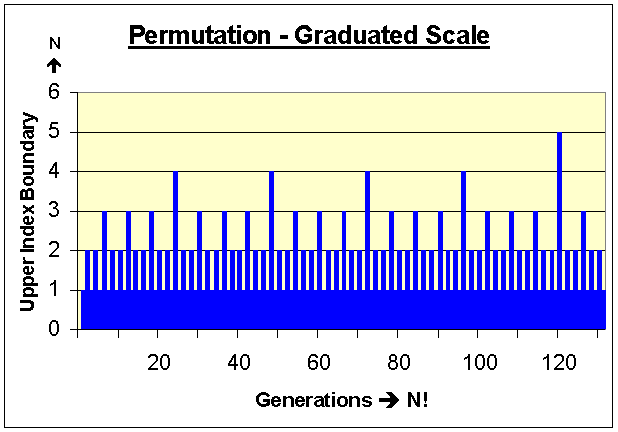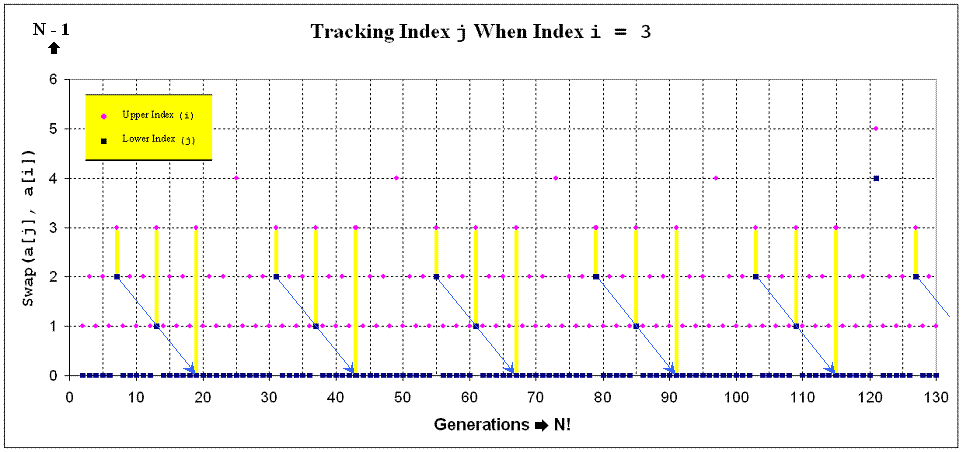# The Countdown QuickPerm Algorithm (Head):(Formally Example_01_Alpha)

QuickPerm - Head Permutations Using a Linear Array Without Recursion (Alpha)
 `````` // NOTICE: Copyright 1991-2008, Phillip Paul Fuchs #define N 12 // number of elements to permute. Let N > 2 void QuickPerm(void) { unsigned int a[N], p[N+1]; register unsigned int i, j, tmp; // Upper Index i; Lower Index j for(i = 0; i < N; i++) // initialize arrays; a[N] can be any type { a[i] = i + 'A'; // a[i] value is not revealed and can be arbitrary p[i] = i; } p[N] = N; // p[N] > 0 controls iteration and the index boundary for i //display(a, 0, 0); // remove comment to display array a[] i = 1; // setup first swap points to be 1 and 0 respectively (i & j) while(i < N) { p[i]--; // decrease index "weight" for i by one j = i % 2 * p[i]; // IF i is odd then j = p[i] otherwise j = 0 tmp = a[j]; // swap(a[j], a[i]) a[j] = a[i]; a[i] = tmp; //display(a, j, i); // remove comment to display target array a[] i = 1; // reset index i to 1 (assumed) while (!p[i]) // while (p[i] == 0) { p[i] = i; // reset p[i] zero value i++; // set new index value for i (increase by one) } // while(!p[i]) } // while(i < N) } // QuickPerm() ``````
QuickPerm Function to Display Permutations (Optional)
 `````` // NOTICE: Copyright 1991-2008, Phillip Paul Fuchs void display(unsigned int *a, unsigned int j, unsigned int i)             { for(unsigned int x = 0; x < N; x++) printf("%c ",a[x]); printf(" swapped(%d, %d)\n", j, i); getch(); // press any key to continue... } // display() ``````
Fundamental Analysis of the QuickPerm Algorithm Above {Switch to Numbers}
 Number of Objectsto Permute (N) a[N] Display ofInitial Sequence a[N] Display ofFinal Sequence Total Number of UniqueCombinations Produced (N!) 3 A B C C B A 2 * 3 = 6 4 A B C D D A B C 6 * 4 = 24 5 A B C D E E B C D A 24 * 5 = 120 6 A B C D E F F C D A B E 120 * 6 = 720 7 A B C D E F G G B C D E F A 720 * 7 = 5040 8 A B C D E F G H H C D E F A B G 5040 * 8 = 40320 9 A B C D E F G H I I B C D E F G H A 40320 * 9 = 362880 if N is even A B C . . . (N - 1) N N C D . . . (N - 2) A B (N - 1) (N - 1)! * N = N! if N is odd A B C . . . (N - 1) N N B C . . . (N - 1) A (N - 1)! * N = N!

A Permutation Ruler:Next is tail permutations represented in EXAMPLE 02.

[]

[] | [] | [] | [] | []
[] | []
[] | [] | []
[]